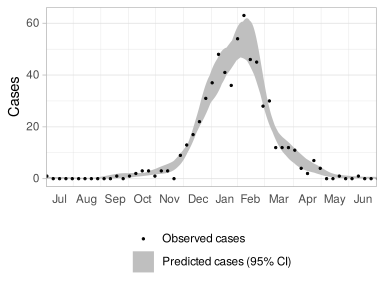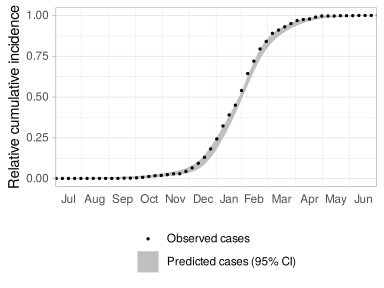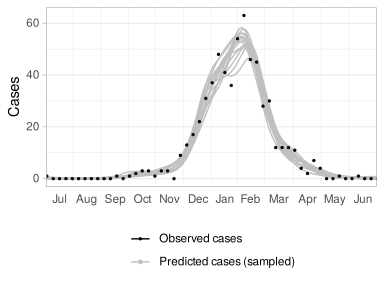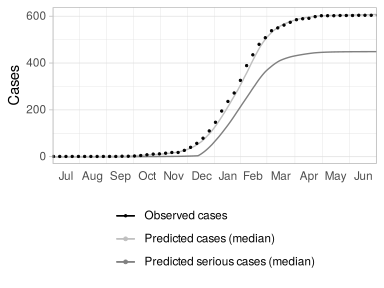# Disease outbreak outcome estimation using penalized splines

## Overview

The purpose of this package is to allow estimation of complex outcome measures of infectious disease outbreaks.

The package works by using generalized additive models (GAMs) with penalized basis splines (P-splines) to approximate the observed data. Approximating splines are sampled from their distribution, and for each approximating spline, the outcome measure of interest is calculated. This yields a sampling distribution of the outcome measure of interest, from which point and itnerval estimates can then be obtained.

We begin by loading the package and a few other libraries needed by the code below:

``````library(pspline.inference)

library(mgcv)
library(magrittr)
library(dplyr)

library(kableExtra)

# Housekeeping for publishing on CRAN
source("cran.R")

# Housekeeping for parallel computation
source("parallel.R")

# Customizations for figures
source("figures.R")``````

## Loading data and setting up the model

Next, we load observations. The example dataset is a CSV file consisting of weekly (`time`) count of cases of a seasonal infectious disease (`cases`).

We also calculate observed cumulative incidence (absolute and relative) here; these are used only for subsequent visualization, not for analysis.

``````obs <- read.csv(system.file("extdata", "seasonal.csv", package="pspline.inference"))
obs\$cases.cum <- cumsum(obs\$cases)
obs\$cases.cumrel <- obs\$cases.cum / max(obs\$cases.cum)``````

Next, we generate a log-linked (`family=poisson`) GAM with 20-knot (`k=20`) cubic (`m=3`) cyclic P-splines (`bs="cp"`). You can vary the number of knots as needed. Fewer knots result in faster computation, but looser fit; more knots will take longer to compute, but increasing the number of knots beyond a certain point will not improve the fit. In your analysis, start with a small number of knots and increase it until adding more doesn’t change the results.

``model <- gam(cases ~ s(time, k=20, bs="cp", m=3), family=poisson, data=obs)``

Generate a vector of time values at which the model will be sampled. This is used for analysis (in particular, for estimation of rates of change and areas under curves) as well as visualization (plotting splines). Higher `sampleFreq` increases the accuracy of results, but also increases computation time.

Here, we set up the time time values so they run from one half time period (inclusive) before the start of the observed times to one half time period (non-inclusive) after the end of the observed times.

``````sampleFreq <- 20
modelTime <- seq(min(obs\$time) - 0.5, max(obs\$time) + 0.5 - 1 / sampleFreq, 1 / sampleFreq)
predictors = data.frame(time=modelTime)``````

This is the number of samples that will be drawn from the outcome distribution. Higher `n` decreases the variance of results, but also increases computation time. 20 is enough for draft analysis and this demo.

``n <- 20``

There are two main types of outcome measures that this package can estimate: time series outcomes (in which an outcome estimate is calculated for each observed time point), and scalar outcomes (in which a single overall outcome estimate is calculated across all time points).

## Example 1: Time series outcome estimation

In this simple example, we estimate the 95% confidence interval for infection case counts. The workhorse of time series estimation is the `pspline.estimate.timeseries` function, which takes three parameters: a data frame of predictors at which the outcome will be estimated (`predictors`, which we set up above), a function which calculates the desired outcome, and the number of samples we want to draw (`n`).

We will see examples later of how to estimate custom outcomes, but for this simple example we can use the `pspline.outbreak.cases` function (which is built into the package) to calculate our outcome of interest.

``````casesEst <- model %>%
pspline.estimate.timeseries(predictors, pspline.outbreak.cases, samples=n, level=0.95)``````

The result of calling `pspline.estimate.timeseries` includes predictors, point estimates of our desired outcome (`median`), as well as an upper and lower confidence level (`upper` and `lower`), which we can plot. The results are what you might expect: the confidence interval is narrow outside of the main part of outbreak, and widest at the outbreak’s peak.

``````ggplot(casesEst, c("casesObs", "casesEst")) +
geom_ribbon(aes(x=time, ymin=cases.lower, ymax=cases.upper, fill="casesEst")) +
geom_point(data=obs, aes(x=time, y=cases, color="casesObs", size="casesObs")) +
labs(x=NULL, y="Cases")``````## Example 2: Time series outcome estimation

Another simple outcome measure that the package can calculate for us is the relative cumulative incidence, using the `pspline.outbreak.cumcases.relative` function. Here we estimate and plot cumulative incidence at the 95% confidence level. Because we are calculating relative incidence, the outcome is constrained to 0 before the outbreak starts and to 1 after the outbreak ends, and therefore the confidence intervals are at their narrowest before and after the outbreak.

``````cumCasesRelEst <- model %>%
pspline.estimate.timeseries(predictors, pspline.outbreak.cumcases.relative, samples=n, level=0.95)``````
``````ggplot(cumCasesRelEst, c("casesObs", "casesEst")) +
geom_ribbon(aes(x=time, ymin=cases.cumrel.lower, ymax=cases.cumrel.upper, fill="casesEst")) +
geom_point(data=obs, aes(x=time, y=cases.cumrel, color="casesObs", size="casesObs")) +
labs(x=NULL, y="Relative cumulative incidence")``````## Example 3: Accessing individual time series samples

The package provides access to the individual samples of the outcome measure, which can be helpful for visualization. For example, if you ask for 15 sampled estimates of case counts, you will get a data frame with a `pspline.sample` column identifying the 15 time series samples:

``````casesSamples <- model %>%
pspline.sample.timeseries(predictors, pspline.outbreak.cases, samples=15)

ggplot(casesSamples, c("casesObs", "casesEstSamples")) +
geom_line(aes(x=time, y=cases, group=pspline.sample, color="casesEstSamples")) +
geom_point(data=obs, aes(x=time, y=cases, color="casesObs", size="casesObs")) +
labs(y="Cases")``````Here `casesSamples` is a data frame of `cases` values for each `time` value, for 100 different samples. The samples are differentiated by the `pspline.sample` column.

## Example 4: Estimation of a custom time series outcome

Suppose that the infection we are investigating progresses to serious disease in 80% of cases, but that it can be treated — before it progresses — with a treatment that has a 90% success rate. Also suppose that our supply is limited to 50 treatments, and that treatment is administered to all infected people until we run out of supply.

Then, among the first 100 cases, 10% will fail treatment, of which 80% will progress to serious disease; for all subsequent cases, no treatment will be available, and 80% will progress to serious disease. We are interested in an estimate of the number of cases of serious disease (as a function of time), based on our observations of the number of cases of the underlying infection.

The `pspline.inference` package does not include a built-in way to calculate this outcome, but we can write our own function to do it. This function will take a model object (`model`, obtained from the call to `gam()` above) and a data frame of predictors (`data`). It will return a data frame of calculated outcome estimates.

``````seriousDiseaseCases <- function(model, data) {
# Get predicted incidence and cumulative incidence for the given param values
cumcases = pspline.outbreak.calc.cumcases(data\$time, data\$cases)

# Calculate when we hit 50 cases
treatmentAvail <- cumcases < 50

# Calculate serious disease case counts -- 80% of (10% before we run out of treatment + 100% after).
seriouscases = data\$cases * 0.8
seriouscases[treatmentAvail] = seriouscases[treatmentAvail] * 0.1

data %>%
mutate(seriouscases.cum=pspline.outbreak.calc.cumcases(time, seriouscases)) %>%
select(-cases)
}``````

We can now combine our custom outcome measure calculation with time series estimation:

``````seriousEst <- model %>%
pspline.estimate.timeseries(predictors, seriousDiseaseCases, samples=n, level=0.95)``````

We’ll also calculate an estimate of all cumulative cases (serious or not), to better visualize the effect of the treatment

``````cumCasesEst <- model %>%
pspline.estimate.timeseries(predictors, pspline.outbreak.cumcases, samples=n, level=0.95)``````

As you might imagine, as the estimated number of all cases rises past 50 and medication becomes unavailable, the estimated number of serious cases takes off sharply.

``````ggplot(seriousEst, c("casesObs", "casesEstMedian", "seriousEstMedian")) +
geom_line(aes(x=time, y=seriouscases.cum.median, color="seriousEstMedian")) +
geom_line(data=cumCasesEst, aes(x=time, y=cases.cum.median, color="casesEstMedian")) +
geom_point(data=obs, aes(x=time, y=cases.cum, color="casesObs", size="casesObs")) +
labs(y="Cases")``````Our custom outcome calculation function would probably be more useful if it allowed progression rate, treatment success rate, and treatment supply to be varied. We can accomplish this by using a function within a function:

``````seriousDiseaseCases <- function(progressionRate, treatmentSuccessRate, treatmentMax) {
function(model, data) {
# Calculate cumulative incidence
cumcases = pspline.outbreak.calc.cumcases(data\$time, data\$cases)

# Calculate when we hit 100 cases
treatmentAvail <- cumcases < treatmentMax

# Calculate serious disease case counts -- 80% of (10% before we run out of treatment + 100% after).
seriouscases = data\$cases * progressionRate
seriouscases[treatmentAvail] = seriouscases[treatmentAvail] * (1 - treatmentSuccessRate)

data %>%
mutate(seriouscases.cum=pspline.outbreak.calc.cumcases(time, seriouscases)) %>%
select(-cases)
}
}``````

This is how we would use it:

``````seriousEst <- model %>%
pspline.estimate.timeseries(
predictors,
seriousDiseaseCases(progressionRate=0.8, treatmentSuccessRate=0.9, treatmentMax=50),
samples=n,
level=0.95
)``````

## Example 5: Scalar outcome measures

Rather than considering the expected incidence of the infection at hand, and its serious manifestation, let us now consider a related question: if we administer preventative medication on a first-come-first-served basis, and we have 50 doses of it, when do we expect to run out?

This is a scalar outcome measure, and we can estimate it using the `pspline.estimate.scalars` function. Similar to `outbreak.estimate.timeseries`, it takes a model, predictors, an outcome calculation function, the number of samples to draw, and the confidence interval. Also similar to `pspline.estimate.timeseries`, it returns median and lower/upper confidence limits for the outcome measure of interest.

For this particular outcome measure, we need to write a function to calculate it. We’ll use the same function-in-function technique to allow us to customize the number of medication doses as needed:

``````medicationSupplyEnd <- function(medicationQuantity) {
function(model, data) {
# Get predicted cumulative incidence for the given param values
data %<>% mutate(
cumcases=pspline.outbreak.calc.cumcases(time, cases)
)

# Find where cumulative incidence exceeds the amount of medication we have
idx = sum(data\$cumcases < medicationQuantity)

# Use the point halfway between latest time below the threshold and the earliest time above the threshold
data.frame(supply.duration=mean(c(data\$time[idx], data\$time[idx + 1])))
}
}

supplyDurationEst <- model %>%
pspline.estimate.scalars(predictors, medicationSupplyEnd(medicationQuantity=50))``````
``````kable_styling(
kable(
supplyDurationEst,
caption="Estimated time before medication supply is exhausted, in weeks since July 1st",
col.names=c("Lower CL", "Median", "Upper CL")
)
)``````
Estimated time before medication supply is exhausted, in weeks since July 1st
Lower CL Median Upper CL
23.025 24.025 24.75125

## Example 6: Accessing individual scalar samples

Similar to time series outcomes, we can obtain the individual samples of a scalar outcome using the `pspline.sample.scalars` function. This is useful for visualization – in this example, we show the density plot of the estimated time when medication supply will run out:

``````supplyDurationSamples <- model %>%
pspline.sample.scalars(predictors, medicationSupplyEnd(medicationQuantity=50), samples=n)

ggplot(seriousEst, c("casesObs", "casesEst", "supplyEnd")) +
geom_ribbon(data=cumCasesEst, aes(x=time, ymin=cases.cum.lower, ymax=cases.cum.upper, fill="casesEst", color="casesEst")) +
geom_point(data=obs, aes(x=time, y=cases.cum, color="casesObs", size="casesObs")) +
geom_density(
data=supplyDurationSamples,
aes(x=supply.duration, y=..density..*50, fill="supplyEnd", color="supplyEnd"),
trim=TRUE
) +
labs(x=NULL, y="Cumulative incidence")``````